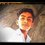# Accelerating Cylinders !!Someone pls help !!

Three identical cylinders are arranged in a triangle(one lying on top of the other two and all 3 are in contact), with the bottom two lying on the ground.The ground and the cylinders are frictionless. You apply a constant horizontal force (directed to the right) on the left cylinder. Let a be the acceleration you give to the system. For what range of a will all three cylinders remain in contact with each other ?Note by Ch Nikhil
5 years, 6 months ago

This discussion board is a place to discuss our Daily Challenges and the math and science related to those challenges. Explanations are more than just a solution — they should explain the steps and thinking strategies that you used to obtain the solution. Comments should further the discussion of math and science.

When posting on Brilliant:

• Use the emojis to react to an explanation, whether you're congratulating a job well done , or just really confused .
• Ask specific questions about the challenge or the steps in somebody's explanation. Well-posed questions can add a lot to the discussion, but posting "I don't understand!" doesn't help anyone.
• Try to contribute something new to the discussion, whether it is an extension, generalization or other idea related to the challenge.

MarkdownAppears as
*italics* or _italics_ italics
**bold** or __bold__ bold
- bulleted- list
• bulleted
• list
1. numbered2. list
1. numbered
2. list
Note: you must add a full line of space before and after lists for them to show up correctly
paragraph 1paragraph 2

paragraph 1

paragraph 2

[example link](https://brilliant.org)example link
> This is a quote
This is a quote
    # I indented these lines
# 4 spaces, and now they show
# up as a code block.

print "hello world"
# I indented these lines
# 4 spaces, and now they show
# up as a code block.

print "hello world"
MathAppears as
Remember to wrap math in $$ ... $$ or $ ... $ to ensure proper formatting.
2 \times 3 $2 \times 3$
2^{34} $2^{34}$
a_{i-1} $a_{i-1}$
\frac{2}{3} $\frac{2}{3}$
\sqrt{2} $\sqrt{2}$
\sum_{i=1}^3 $\sum_{i=1}^3$
\sin \theta $\sin \theta$
\boxed{123} $\boxed{123}$

Sort by:

At the time when the upper cylinder just leaves the contact with the bottom cylinder.

N Sin60 = mg;
N cos60 = ma;
a = g cot60 = g $\frac{1}{\sqrt3}$

- 5 years, 6 months ago

- 5 years, 6 months ago

Hi Nikhil!img

It's is obvious that the cylinder at the top will first loose contact with cylinder at right. The moment it looses contact with that the normal force acting between them would be zero. If we consider a frame of reference which is moving with the CoM of the sytem then pseudo force on top cylinder is $ma$.

So

$N_{1}cos(30)=mg$....(1)

and $N_{1}sin(30)=ma$......(2)

Solving this we get a value of ,say $a_{0}$. So the cylinders would loose contact for all accelerations greater than $a_{0}$.

- 5 years, 6 months ago

Hi Satvik, Can you tell me how you draw the diagram and upload it..??

- 5 years, 6 months ago

Hi Sir!! I draw these diagrams using paint.

First upload the diagram on imgur. Then type:

! [ anything] ( url of the image). Without spaces. :)

- 5 years, 6 months ago

Thanks.... ;)

- 5 years, 6 months ago

You are welcome, Sir! :)

- 5 years, 6 months ago

Thanks a lot @satvik pandey !!

- 5 years, 6 months ago

You are welcome!

- 5 years, 6 months ago